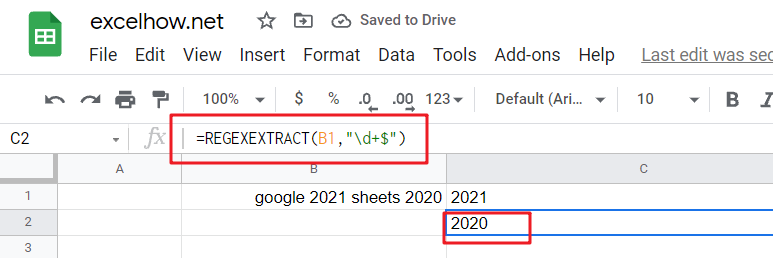To pad a number with zeros, you need to add leading spaces and/or carriers. For example, if the original digit contains 2 digits, three additional characters will be added on before it. If there are 3 digits in total, allocating two places for these extra pieces of information ensures that your output looks correct without having any factual inaccuracies within them!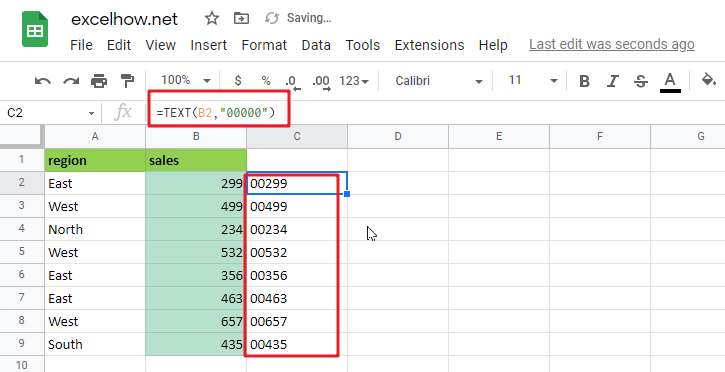Two ways to solve this problem: you can either convert the number into text with leading zeros or apply a custom format that displays numbers As desired. The best approach depends on your application’s needs and how much flexibility there will be in future renovations to accommodate new needs. If converting all numbers back from their textual representation would entail too much work for someone else who might come along later down the line, then it may actually make more sense optically employing an option where some values remain numeric-although not necessarily negative, though!

### Convert To Text Using TEXT Function

Leading zeros can be added easily by using the TEXT function. For example, if you want five characters total and use “00000” as your number format in google sheets, it will return those values after conversion from numbers into text with that specific style!

`=TEXT(B2,"00000")   `With the text “00299”, google sheets automatically aligns it to the left, but you can set alignment manually as you want. To replace original numbers with converted values: copy them first and then select all occurrences of this string in your workbook before pressing Paste Special > Values which will convert those contents into an entry on its own without changing anything else around it, including spacing or font size – very useful if there are many instances!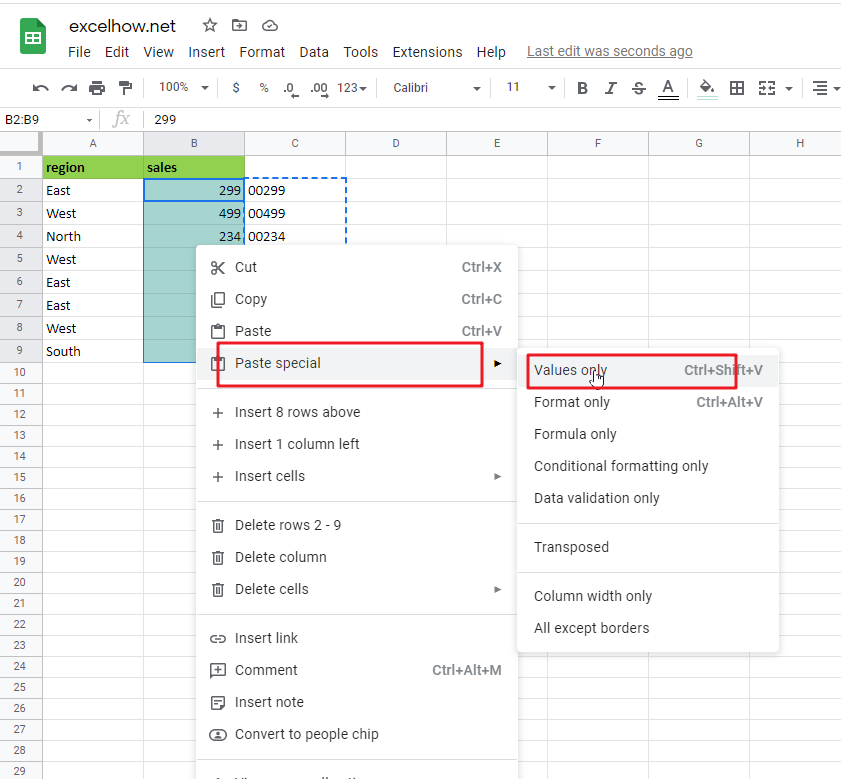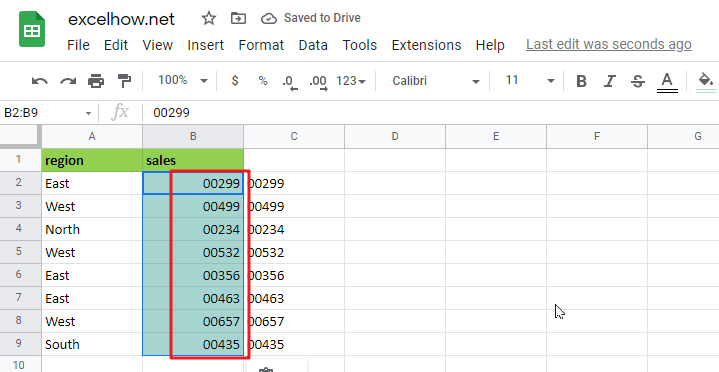### Related Functions

The google sheets TEXT function converts a numeric value into text string with a specified format. The TEXT function is a build-in function in Microsoft Excel and it is categorized as a Text Function. The syntax of the TEXT function is as below: = TEXT (value, Format code)…

This post will guide you how to use Google Sheets TEXT function with syntax and examples.

## Description

The Google Sheets TEXT function converts a numeric value into text string with a specified format.

The TEXT function can be used to convert a number formatted as text according to a specified format in google sheets.  The purpose of this function is to convert a number to text in a number format. and its returned value is a number as text in the given format.

The TEXT function is a build-in function in Google Sheets and it is categorized as a Text Function.

## Syntax

The syntax of the TEXT function is as below:

= TEXT (value, Format code)

Where the TEXT function arguments are:

• number -This is a required argument. The value you want to format.
• Format code– This is a required argument. The format code that you want to apply.

The “`Format Code`” can be used in the excel Text function are shown in the below table.

 Format Code Description Examples 0 only display digits in its place #.00 – Forces the function to display two decimal places =Text(34.234,”\$##.00″) result: \$34.23 # Display the placeholder =Text(4.527,”#.##) result: 4.53 . the position of Decimal Point =Text(342.2,”0.00″) result: 342.20 d Day of the month or day of week d- one or two digit number (1-31) dd- two digit number (01-31) ddd-abbreviated day of week (Mon to Sun) dddd-full name of day of week(Monday to Sunnday) =Text(TODAY(),”DDDD”) result: Monday m The Month of the Year m- one or two digit number mm-two digit number mmm-abbreviated month(Jan to Dec) mmmm-full name of month(January to December)) =Text(TODAY(),”MM/DD/YY”) result:11/06/17 y year yy-two digit representation of year(e.g.01,17) yyyy-four digit representation of year(e.g. 2001,2017) =Text(TODAY(),”MM/DD/YY”) result:11/06/17 h Hour h-one or two digit number (e.g. 1,23) hh-two digit number (e.g. 01,23) =Text(14:16,”hh:mm”) result: 14:16 m Minute m-one or two digit representation (e.g. 1,59) mm-two digit representation (e.g. 01,59) =Text(14:16,”hh:mm”) result: 14:16 s Second s-one or two digit representation (e.g. 1,59) ss=two digit representation (e.g. 01,59)

Important Notes:

• The Text formula converts a numeric value to formatted text, but its result can not be used for calculation purpose in the other excel functions or formula.
• the “format code must be marked in the quotation marks. or it will return “#NAME?” error.
• the asterisk character cannot be used in format code.
• The TEXT function takes two arguments, `value` and `format_code`. `Value` is the number, date or time to format. and it should be a numeric value. `Format_code` is a pattern by which to format the number.
• The output of TEXT function is always a text string. If you want to format a number, just apply a regular number formatting in google sheets.

## Google Sheets TEXT Function Examples

The below examples will show you how to use google sheets TEXT function to convert a numeric value into a text string with specified format code.

#1 To convert a numeric value(34293) with MM/DD/YY format code in B1 cell, just using formula:

`= TEXT(B1,"MM/DD/YY") //it returns 11/20/93`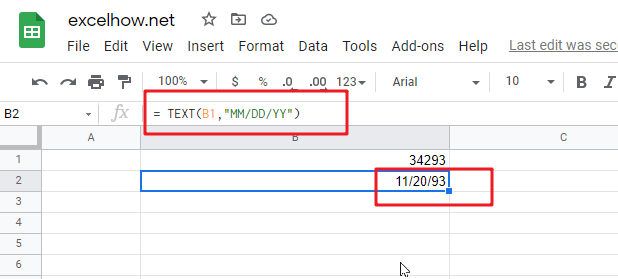This post will guide you how to use Google Sheets REGEXREPLACE function with syntax and examples.

## Description

The Google Sheets REGEXREPLACE function is to replace a part of a text string with a different substring using regular expressions.

The REGEXREPLACE function can be used to replace a substring from a text string according to a given regular expression in google sheets.

The REGEXREPLACE function is a build-in function in Google Sheets and it is categorized as a Text Function. And The REGEXREPLACE function is one of the three REGEX functions (REGEXEXTRACT, REGEXMATCH and REGEXREPLACE )in google sheets.

## Syntax

The syntax of the REGEXREPLACE function is as below:

=REGEXREPLACE (text, regular_expression, replacement)

Where the REGEXREPLACE function arguments are:

• text -This is a required argument. The text string that you want to extract a substring based on regular expression.
• regular_expression – This is a required argument. The `Regular_expression` is a regular expression. It can be used to match the part of the text that you want to extract. The regular expression argument should be entered in double-quote characters. And `Regular_expression` is a search patterns that allow you to find a certain patterns in original text string.
• Replacement – The text which will be inserted into the original text.

Note:

• The REGEXREPLACE function takes two arguments. It checks whether a piece of text matches a given regular expression.
• The REGEXREPLACE function only works with text value as its first argument and returns a text result. If you wish to return a numeric value, and you need to use VALUE function in combination with the REGEXREPLACE function. If the input value is a number, and you can use the TEXT function to convert them.
• Google Sheets supports RE2 for regular expressions.

## Google Sheets REGEXREPLACE Function Examples

The below examples will show you how to use google sheets REGEXREPLACE function to replace a substring from a text according to a regular expression.

#1 Replace all “google” keywords with “mygoogle” text string, and you can use the REGEXREPLACE function with a regular expression “google”, type:

`=REGEXREPLACE(B1,"google","mygoogle") //it returns “mygoogle 2021 sheets 2020”`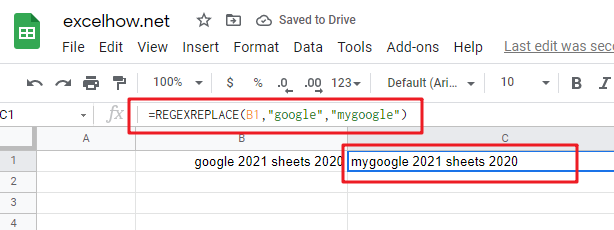#2 Replace all word with “mygoogle” substring from a string in cell B1, and you can use the REGEXREPLACE function with a regular expression “\w+”, type:

`=REGEXREPLACE(B1,"\w+","mygoogle") //it returns “mygoogle mygoogle mygoogle mygoogle”`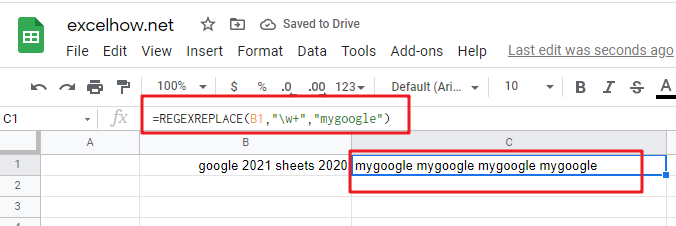#3 Replace the last whole word from a string using REGEXREPLACE function with a regular expression “\w+\$”, type:

`=REGEXREPLACE(B1,"\w+\$","mygoogle") // it returns “google 2021 sheets mygoogle”`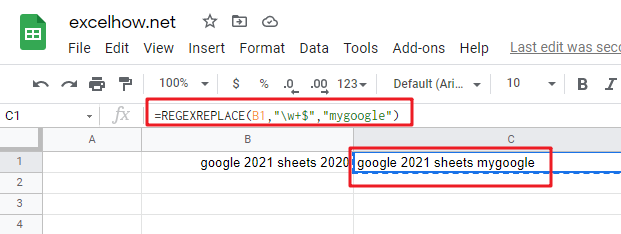#4 Replace all numbers with “2025” from a string in cell B1 and you can use the REGEXREPLACE function with a regular expression “\d+”, type:

`=REGEXREPLACE(B1,"\d+","2025") // it returns “google 2025 sheets 2025”`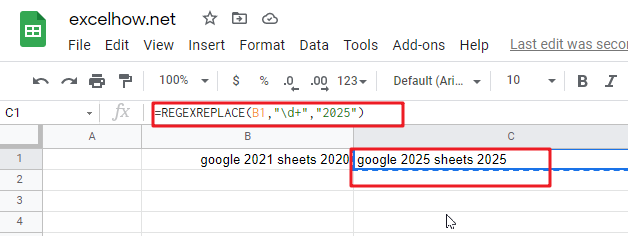#5 Replate the last number with “2025” number from a string in cell B1, and you can use the REGEXREPLACE function with a regular expression “\d+\$”, type:

`=REGEXREPLACE(B1,"\d+\$","2025") // it returns “google 2021 sheets 2025”`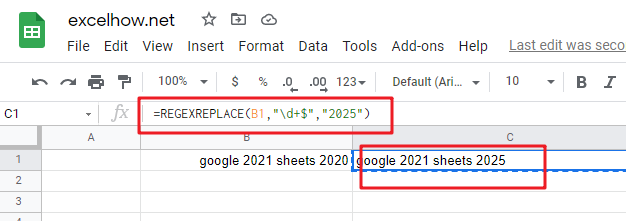This post will guide you how to use Google Sheets REGEXMATCH function with syntax and examples.

## Description

The Google Sheets REGEXMATCH function is to check whether a piece of text matches a regular expression.

The REGEXMATCH function can be used to check whether a substring from a text string matches a given regular expression in google sheets. And it returns a logic value, TRUE or FALSE.

The REGEXMATCH function is a build-in function in Google Sheets and it is categorized as a Text Function.

## Syntax

The syntax of the REGEXMATCH function is as below:

= REGEXMATCH (text, regular_expression)

Where the REGEXMATCH function arguments are:

• text -This is a required argument. The text string that you want to extract a substring based on regular expression.
• regular_expression – This is a required argument. The Regular_expression is a regular expression. It can be used to match the part of the text that you want to extract. The regular expression argument should be entered in double-quote characters.

Note:

• The REGEXMATCH function takes two arguments. It checks whether a piece of text matches a given regular expression.
• The REGEXMATCH function only works with text value as its first argument and returns a text result. If you wish to return a numeric value, and you need to use VALUE function in combination with the REGEXEXTRACT function. If the input value is a number, and you can use the TEXT function to convert them.
• Google Sheets supports RE2 for regular expressions.

## Google Sheets REGEXMATCH Function Examples

The below examples will show you how to use google sheets REGEXMATCH function to extract a substring from a text according to a regular expression.

#1 Check if text value in Cell B1 matches a given regular expression, using the REGEXMATCH function with a regular expression “google” , type:

`= REGEXMATCH (B1,"google") //it returns “TRUE”`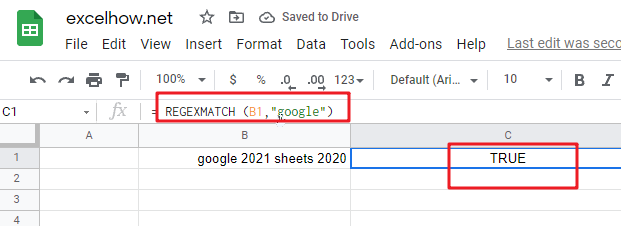#2 Check if text value in cell B1 matches either of the words in a sentence using REGEXMATCH function with a regular expression “google|sheets” , type:

`=REGEXMATCH(B1,"google|sheets") // it returns “TRUE”`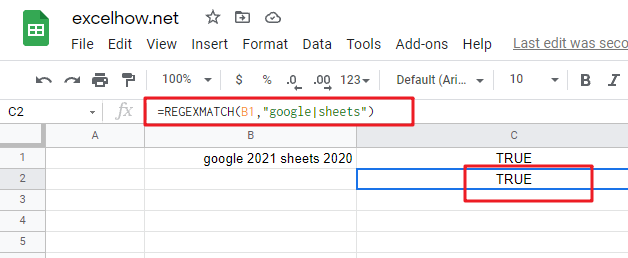#3 Check if text value in Cell B1 matches any number of decimal digits using REGEXMATCH function with a regular expression “\d+” , type:

`= REGEXMATCH(B1,"\d+") // it returns “TRUE”`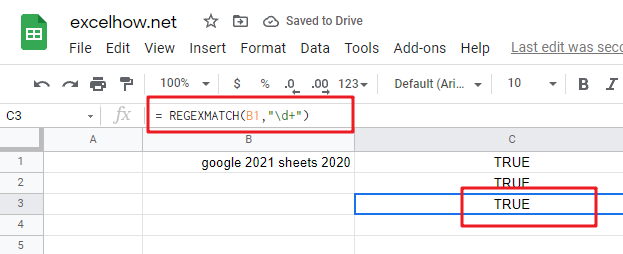#4 Check if text value in Cell B1 matches any specific decimal digits using REGEXMATCH function with a regular expression “[2-3]” , type:

`= REGEXMATCH(B1,"[2-3]") // it returns “TRUE”`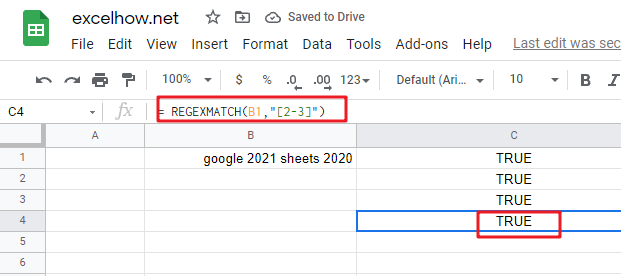This post will guide you how to use Google Sheets REGEXEXTRACT function with syntax and examples.

## Description

The Google Sheets REGEXEXTRACT function uses a regular expression to extract the first matching substring.

The REGEXEXTRACT function can be used to extract the first matching substring from a text string according to a given regular expression in google sheets. So it returns the part of the specified string that matches the given pattern in a regular expression.

The REGEXEXTRACT function is a build-in function in Google Sheets and it is categorized as a Text Function.

## Syntax

The syntax of the REGEXEXTRACT function is as below:

=REGEXEXTRACT (text, regular_expression)

Where the REGEXEXTRACT function arguments are:

• text -This is a required argument. The text string that you want to extract a substring based on regular expression.
• regular_expression – This is a required argument. The `Regular_expression` is a regular expression. It can be used to match the part of the text that you want to extract. The regular expression argument should be entered in double-quote characters.

Note:

• The REGEXEXTRACT function takes two arguments. It extracts the first matching substrings according to a given regular expression.
• The REGEXEXTRACT function also can return multiple results with capture groups. A capture group is also a part of a regular expression that can be enclosed in parentheses.
• The REGEXEXTRACT function only works with text value as its first argument and returns a text result. If you wish to return a numeric value, and you need to use VALUE function in combination with the REGEXEXTRACT function. If the input value is a number, and you can use the TEXT function to convert them.
• Google Sheets supports RE2 for regular expressions.

## Google Sheets REGEXEXTRACT Function Examples

The below examples will show you how to use google sheets REGEXEXTRACT function to extract a substring from a text according to a regular expression.

#1 Extract the first 5 characters from a string using REGEXEXTRACT function with a regular expression “…..” , type:

`=REGEXEXTRACT(B1,".....") //it returns “googl”`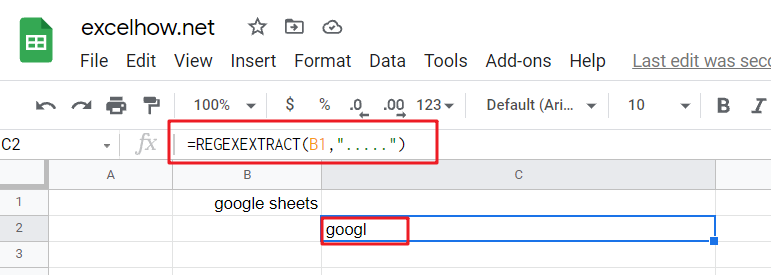#2 Extract the last 5 characters from a string using REGEXEXTRACT function with a regular expression “…..\$” , type:

`=REGEXEXTRACT(B1,".....\$") // it returns “heets”`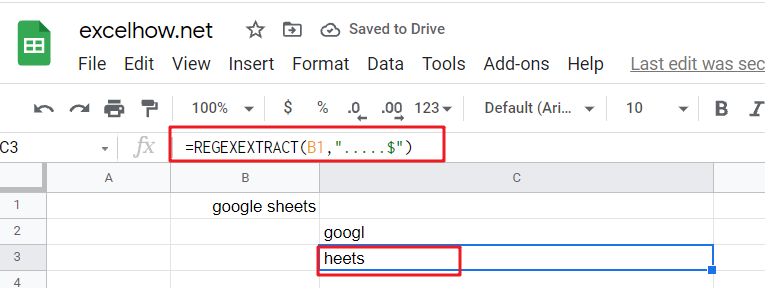#3 Extract the first whole word from a string using REGEXEXTRACT function with a regular expression “\w+” , type:

`=REGEXEXTRACT(B1,"\w+") // it returns “google”`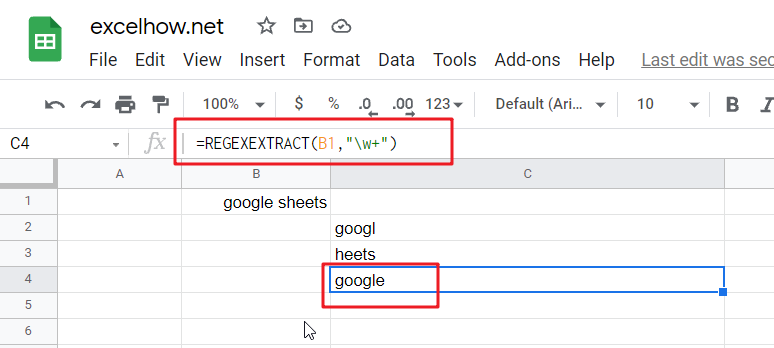#4 Extract the last whole word from a string using REGEXEXTRACT function with a regular expression “\w+\$” , type:

`=REGEXEXTRACT(B1,"\w+\$") // it returns “sheets”`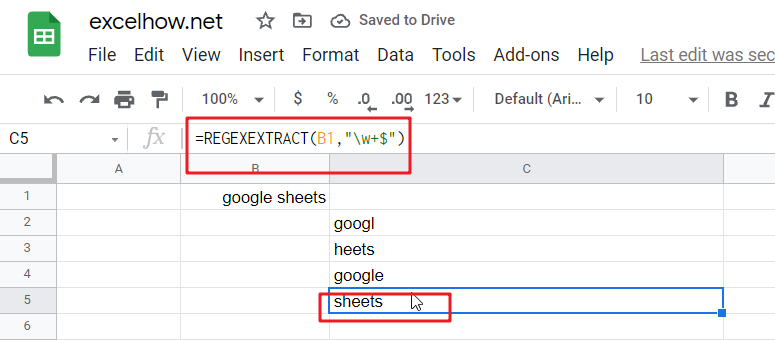#5 Extract the first number from a string using REGEXEXTRACT function with a regular expression “\d+” , type:

`=REGEXEXTRACT(B1,"\d+") // it returns “2021”`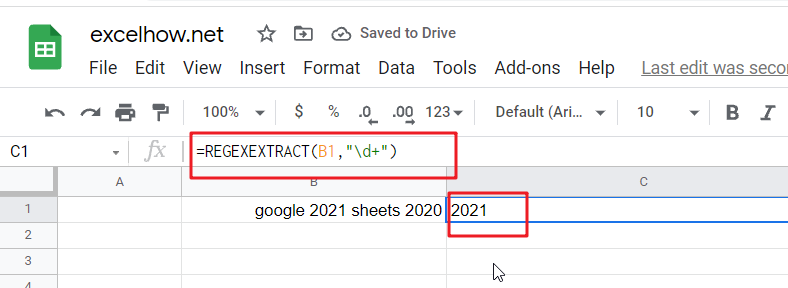#6 Extract the last number from a string using REGEXEXTRACT function with a regular expression “\d+\$” , type:

`=REGEXEXTRACT(B1,"\d+") // it returns “2020”`